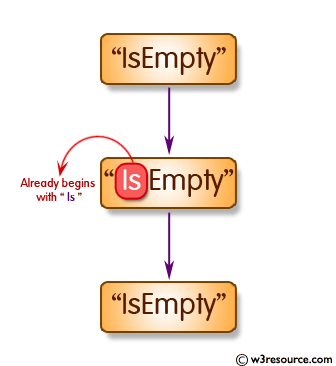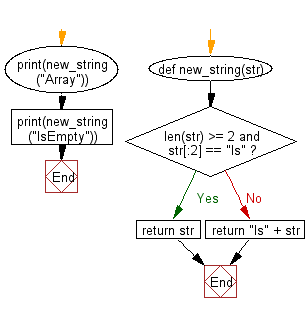﻿ Python: Get a new string from a given string where 'Is' has been added to the front. If the given string already begins with 'Is' then return the string unchanged - w3resource# Python: Get a new string from a given string where 'Is' has been added to the front. If the given string already begins with 'Is' then return the string unchanged

## Python Basic: Exercise-19 with Solution

Write a Python program to get a new string from a given string where "Is" has been added to the front. If the given string already begins with "Is" then return the string unchanged.

Pictorial Presentation:Sample Solution:-

Python Code:

``````def new_string(str):
if len(str) >= 2 and str[:2] == "Is":
return str
return "Is" + str

print(new_string("Array"))
print(new_string("IsEmpty"))
```
```

Sample Output:

```IsArray
IsEmpty
```

Flowchart:## Visualize Python code execution:

The following tool visualize what the computer is doing step-by-step as it executes the said program:

Python Code Editor:

Have another way to solve this solution? Contribute your code (and comments) through Disqus.

What is the difficulty level of this exercise?

Test your Python skills with w3resource's quiz

﻿

## Python: Tips of the Day

Unpack variables from iterable:

```# One can unpack all iterables (tuples, list etc)
>>> a, b, c = 1, 2, 3
>>> a, b, c
(1, 2, 3)

>>> a, b, c = [1, 2, 3]
>>> a, b, c
(1, 2, 3)
```